Pulkit Sharma — May 27, 2019

Introduction

It is crucial to understand customer behavior in any industry. I realized this last year when my chief marketing officer asked me – “Can you tell me which existing customers should we target for our new product?”

That was quite a learning curve for me. I quickly realized as a data scientist how important it is to segment customers so my organization can tailor and build targeted strategies. This is where the concept of clustering came in ever so handy!

Problems like segmenting customers are often deceptively tricky because we are not working with any target variable in mind. We are officially in the land of unsupervised learning where we need to figure out patterns and structures without a set outcome in mind. It’s both challenging and thrilling as a data scientist.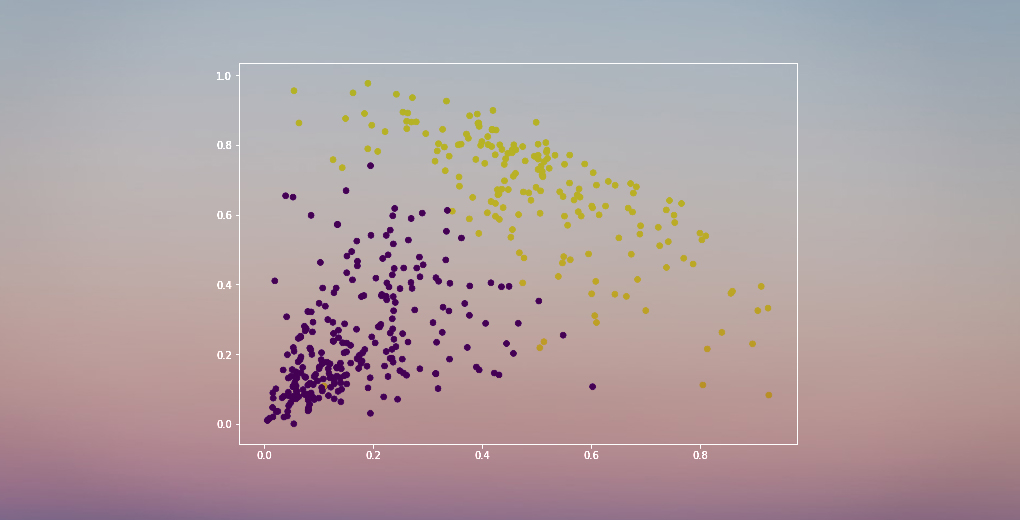Now, there are a few different ways to perform clustering (as you’ll see below). I will introduce you to one such type in this article – hierarchical clustering.

We will learn what hierarchical clustering is, its advantage over the other clustering algorithms, the different types of hierarchical clustering and the steps to perform it. We will finally take up a customer segmentation dataset and then implement hierarchical clustering in Python. I love this technique and I’m sure you will too after this article!

Note: As mentioned, there are multiple ways to perform clustering. I encourage you to check out our awesome guide to the different types of clustering:

To learn more about clustering and other machine learning algorithms (both supervised and unsupervised) check out the following comprehensive program-

1. Supervised vs Unsupervised Learning
2. Why Hierarchical Clustering?
3. What is Hierarchical Clustering?
4. Types of Hierarchical Clustering
1. Agglomerative Hierarchical Clustering
2. Divisive Hierarchical Clustering
5. Steps to perform Hierarchical Clustering
6. How to Choose the Number of Clusters in Hierarchical Clustering?
7. Solving a Wholesale Customer Segmentation Problem using Hierarchical Clustering

Supervised vs Unsupervised Learning

It’s important to understand the difference between supervised and unsupervised learningunsupervised learning before we dive into hierarchical clustering. Let me explain this difference using a simple example.

Suppose we want to estimate the count of bikes that will be rented in a city every day: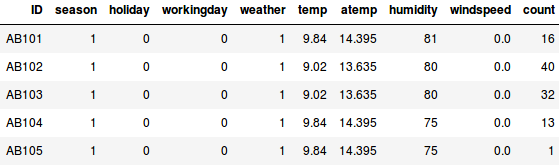Or, let’s say we want to predict whether a person on board the Titanic survived or not: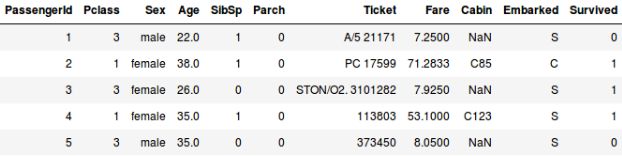We have a fixed target to achieve in both these examples:

• In the first example, we have to predict the count of bikes based on features like the season, holiday, workingday, weather, temp, etc.
• We are predicting whether a passenger survived or not in the second example. In the ‘Survived’ variable, 0 represents that the person did not survive and 1 means the person did make it out alive. The independent variables here include Pclass, Sex, Age, Fare, etc.

So, when we are given a target variable (count and Survival in the above two cases) which we have to predict based on a given set of predictors or independent variables (season, holiday, Sex, Age, etc.), such problems are called supervised learning problems.

Let’s look at the figure below to understand this visually: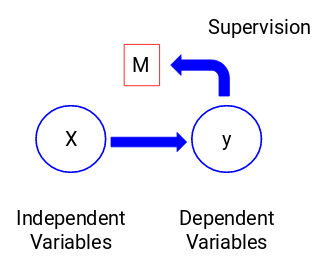Here, y is our dependent or target variable, and X represents the independent variables. The target variable is dependent on X and hence it is also called a dependent variable. We train our model using the independent variables in the supervision of the target variable and hence the name supervised learning.

Our aim, when training the model, is to generate a function that maps the independent variables to the desired target. Once the model is trained, we can pass new sets of observations and the model will predict the target for them. This, in a nutshell, is supervised learning.

There might be situations when we do not have any target variable to predict. Such problems, without any explicit target variable, are known as unsupervised learning problems. We only have the independent variables and no target/dependent variable in these problems.We try to divide the entire data into a set of groups in these cases. These groups are known as clusters and the process of making these clusters is known as clustering.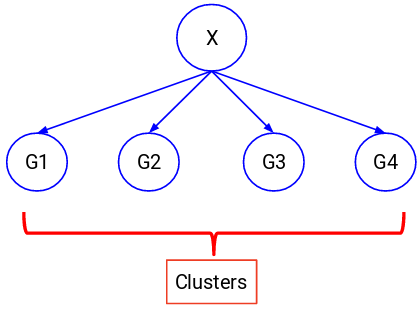This technique is generally used for clustering a population into different groups. A few common examples include segmenting customers, clustering similar documents together, recommending similar songs or movies, etc.

There are a LOT more applications of unsupervised learning. If you come across any interesting application, feel free to share them in the comments section below!

Now, there are various algorithms that help us to make these clusters. The most commonly used clustering algorithms are K-means and Hierarchical clustering.

Why Hierarchical Clustering?

We should first know how K-means works before we dive into hierarchical clustering. Trust me, it will make the concept of hierarchical clustering all the more easier.

Here’s a brief overview of how K-means works:

1. Decide the number of clusters (k)
2. Select k random points from the data as centroids
3. Assign all the points to the nearest cluster centroid
4. Calculate the centroid of newly formed clusters
5. Repeat steps 3 and 4

It is an iterative process. It will keep on running until the centroids of newly formed clusters do not change or the maximum number of iterations are reached.

But there are certain challenges with K-means. It always tries to make clusters of the same size. Also, we have to decide the number of clusters at the beginning of the algorithm. Ideally, we would not know how many clusters should we have, in the beginning of the algorithm and hence it a challenge with K-means.

This is a gap hierarchical clustering bridges with aplomb. It takes away the problem of having to pre-define the number of clusters. Sounds like a dream! So, let’s see what hierarchical clustering is and how it improves on K-means.

What is Hierarchical Clustering?

Let’s say we have the below points and we want to cluster them into groups: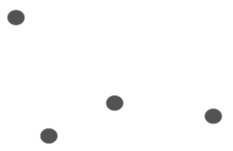We can assign each of these points to a separate cluster: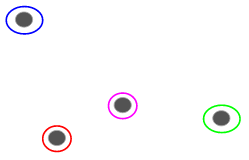Now, based on the similarity of these clusters, we can combine the most similar clusters together and repeat this process until only a single cluster is left: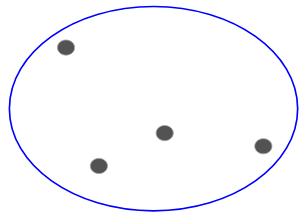We are essentially building a hierarchy of clusters. That’s why this algorithm is called hierarchical clustering. I will discuss how to decide the number of clusters in a later section. For now, let’s look at the different types of hierarchical clustering.

Types of Hierarchical Clustering

There are mainly two types of hierarchical clustering:

1. Agglomerative hierarchical clustering
2. Divisive Hierarchical clustering

Let’s understand each type in detail.

Agglomerative Hierarchical Clustering

We assign each point to an individual cluster in this technique. Suppose there are 4 data points. We will assign each of these points to a cluster and hence will have 4 clusters in the beginning:Then, at each iteration, we merge the closest pair of clusters and repeat this step until only a single cluster is left: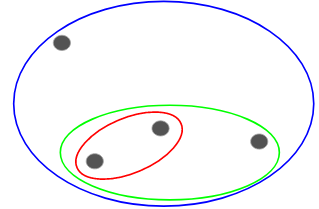We are merging (or adding) the clusters at each step, right? Hence, this type of clustering is also known as additive hierarchical clustering.

Divisive Hierarchical Clustering

Divisive hierarchical clustering works in the opposite way. Instead of starting with n clusters (in case of n observations), we start with a single cluster and assign all the points to that cluster.

So, it doesn’t matter if we have 10 or 1000 data points. All these points will belong to the same cluster at the beginning:Now, at each iteration, we split the farthest point in the cluster and repeat this process until each cluster only contains a single point:We are splitting (or dividing) the clusters at each step, hence the name divisive hierarchical clustering.

Agglomerative Clustering is widely used in the industry and that will be the focus in this article. Divisive hierarchical clustering will be a piece of cake once we have a handle on the agglomerative type.

Steps to Perform Hierarchical Clustering

We merge the most similar points or clusters in hierarchical clustering – we know this. Now the question is – how do we decide which points are similar and which are not? It’s one of the most important questions in clustering!

Here’s one way to calculate similarity – Take the distance between the centroids of these clusters. The points having the least distance are referred to as similar points and we can merge them. We can refer to this as a distance-based algorithm as well (since we are calculating the distances between the clusters).

In hierarchical clustering, we have a concept called a proximity matrix. This stores the distances between each point. Let’s take an example to understand this matrix as well as the steps to perform hierarchical clustering.

Setting up the ExampleSuppose a teacher wants to divide her students into different groups. She has the marks scored by each student in an assignment and based on these marks, she wants to segment them into groups. There’s no fixed target here as to how many groups to have. Since the teacher does not know what type of students should be assigned to which group, it cannot be solved as a supervised learning problem. So, we will try to apply hierarchical clustering here and segment the students into different groups.

Let’s take a sample of 5 students: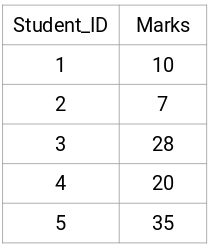Creating a Proximity Matrix

First, we will create a proximity matrix which will tell us the distance between each of these points. Since we are calculating the distance of each point from each of the other points, we will get a square matrix of shape n X n (where n is the number of observations).

Let’s make the 5 x 5 proximity matrix for our example: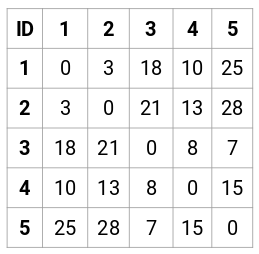The diagonal elements of this matrix will always be 0 as the distance of a point with itself is always 0. We will use the Euclidean distance formula to calculate the rest of the distances. So, let’s say we want to calculate the distance between point 1 and 2:

√(10-7)^2 = √9 = 3

Similarly, we can calculate all the distances and fill the proximity matrix.

Steps to Perform Hierarchical Clustering

Step 1: First, we assign all the points to an individual cluster: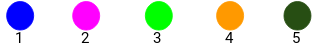Different colors here represent different clusters. You can see that we have 5 different clusters for the 5 points in our data.

Step 2: Next, we will look at the smallest distance in the proximity matrix and merge the points with the smallest distance. We then update the proximity matrix: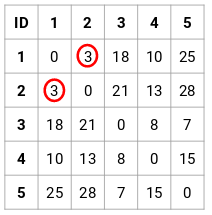Here, the smallest distance is 3 and hence we will merge point 1 and 2: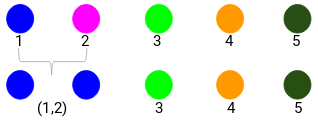Let’s look at the updated clusters and accordingly update the proximity matrix: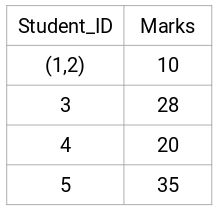Here, we have taken the maximum of the two marks (7, 10) to replace the marks for this cluster. Instead of the maximum, we can also take the minimum value or the average values as well. Now, we will again calculate the proximity matrix for these clusters: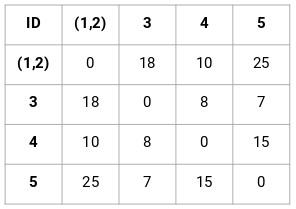Step 3: We will repeat step 2 until only a single cluster is left.

So, we will first look at the minimum distance in the proximity matrix and then merge the closest pair of clusters. We will get the merged clusters as shown below after repeating these steps: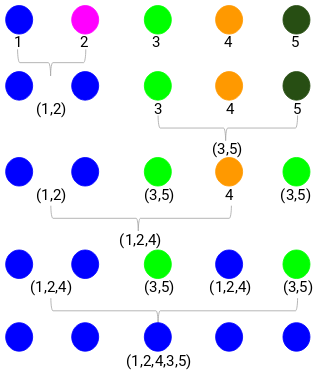We started with 5 clusters and finally have a single cluster. This is how agglomerative hierarchical clustering works. But the burning question still remains – how do we decide the number of clusters? Let’s understand that in the next section.

How should we Choose the Number of Clusters in Hierarchical Clustering?

Ready to finally answer this question that’s been hanging around since we started learning? To get the number of clusters for hierarchical clustering, we make use of an awesome concept called a Dendrogram.

A dendrogram is a tree-like diagram that records the sequences of merges or splits.

Let’s get back to our teacher-student example. Whenever we merge two clusters, a dendrogram will record the distance between these clusters and represent it in graph form. Let’s see how a dendrogram looks like: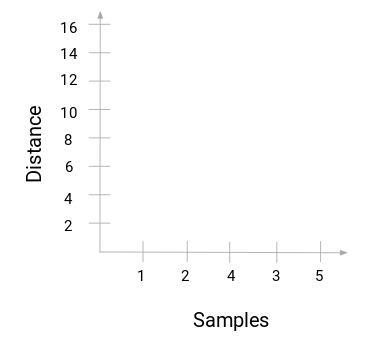We have the samples of the dataset on the x-axis and the distance on the y-axis. Whenever two clusters are merged, we will join them in this dendrogram and the height of the join will be the distance between these points. Let’s build the dendrogram for our example:Take a moment to process the above image. We started by merging sample 1 and 2 and the distance between these two samples was 3 (refer to the first proximity matrix in the previous section). Let’s plot this in the dendrogram: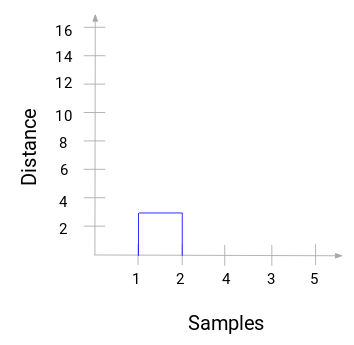Here, we can see that we have merged sample 1 and 2. The vertical line represents the distance between these samples. Similarly, we plot all the steps where we merged the clusters and finally, we get a dendrogram like this: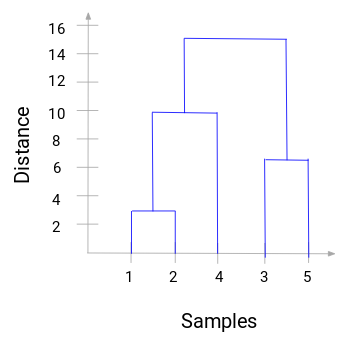We can clearly visualize the steps of hierarchical clustering. More the distance of the vertical lines in the dendrogram, more the distance between those clusters.

Now, we can set a threshold distance and draw a horizontal line (Generally, we try to set the threshold in such a way that it cuts the tallest vertical line). Let’s set this threshold as 12 and draw a horizontal line: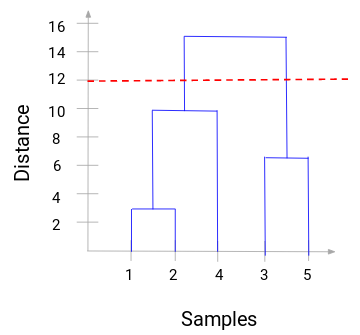The number of clusters will be the number of vertical lines which are being intersected by the line drawn using the threshold. In the above example, since the red line intersects 2 vertical lines, we will have 2 clusters. One cluster will have a sample (1,2,4) and the other will have a sample (3,5). Pretty straightforward, right?

This is how we can decide the number of clusters using a dendrogram in Hierarchical Clustering. In the next section, we will implement hierarchical clustering which will help you to understand all the concepts that we have learned in this article.

Solving the Wholesale Customer Segmentation problem using Hierarchical Clustering

Time to get our hands dirty in Python!

We will be working on a wholesale customer segmentation problem. You can download the dataset using this link. The data is hosted on the UCI Machine Learning repository. The aim of this problem is to segment the clients of a wholesale distributor based on their annual spending on diverse product categories, like milk, grocery, region, etc.

Let’s explore the data first and then apply Hierarchical Clustering to segment the clients.

We will first import the required libraries:

Load the data and look at the first few rows: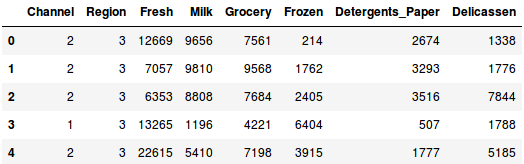There are multiple product categories – Fresh, Milk, Grocery, etc. The values represent the number of units purchased by each client for each product. Our aim is to make clusters from this data that can segment similar clients together. We will, of course, use Hierarchical Clustering for this problem.

But before applying Hierarchical Clustering, we have to normalize the data so that the scale of each variable is the same. Why is this important? Well, if the scale of the variables is not the same, the model might become biased towards the variables with a higher magnitude like Fresh or Milk (refer to the above table).

So, let’s first normalize the data and bring all the variables to the same scale: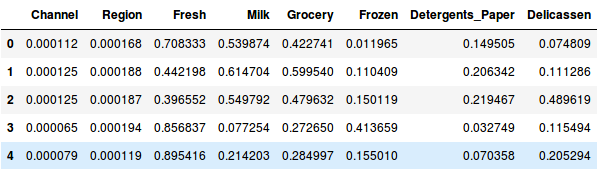Here, we can see that the scale of all the variables is almost similar. Now, we are good to go. Let’s first draw the dendrogram to help us decide the number of clusters for this particular problem: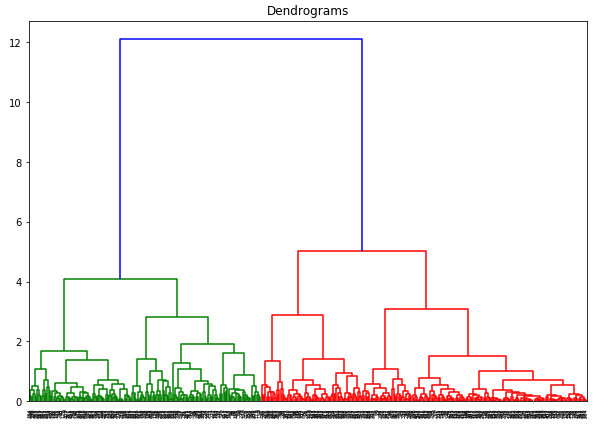The x-axis contains the samples and y-axis represents the distance between these samples. The vertical line with maximum distance is the blue line and hence we can decide a threshold of 6 and cut the dendrogram: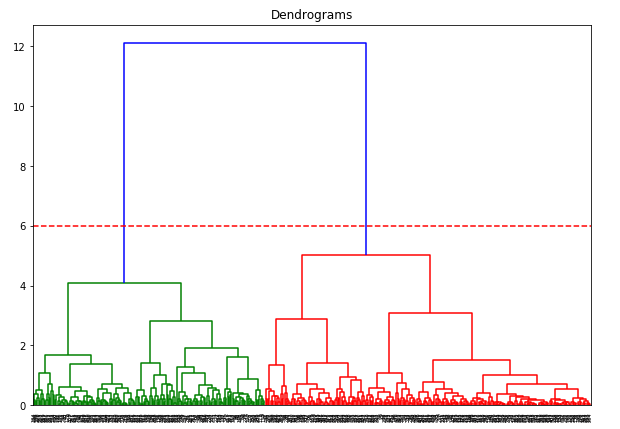We have two clusters as this line cuts the dendrogram at two points. Let’s now apply hierarchical clustering for 2 clusters: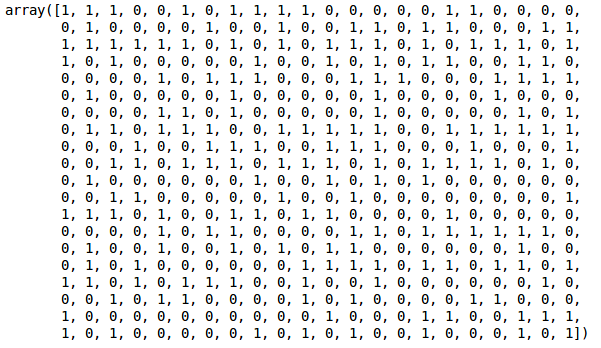We can see the values of 0s and 1s in the output since we defined 2 clusters. 0 represents the points that belong to the first cluster and 1 represents points in the second cluster. Let’s now visualize the two clusters: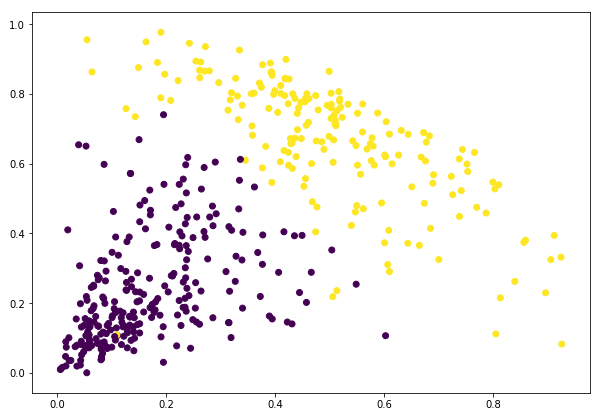Awesome! We can clearly visualize the two clusters here. This is how we can implement hierarchical clustering in Python.

End Notes

Hierarchical clustering is a super useful way of segmenting observations. The advantage of not having to pre-define the number of clusters gives it quite an edge over k-Means.

If you are still relatively new to data science, I highly recommend taking the Applied Machine Learning course. It is one of the most comprehensive end-to-end machine learning courses you will find anywhere. Hierarchical clustering is just one of a diverse range of topics we cover in the course.

What are your thoughts on hierarchical clustering? Do you feel there’s a better way to create clusters using less computational resources? Connect with me in the comments section below and let’s discuss!Pulkit Sharma

My research interests lies in the field of Machine Learning and Deep Learning. Possess an enthusiasm for learning new skills and technologies.

33 thoughts on "A Beginner’s Guide to Hierarchical Clustering and how to Perform it in Python"Prabhakar Krishnamurthy says:May 27, 2019 at 12:45 pm
Dear Pulakit Sharma, Thank you very much for your article. Is it possible to use R to get the same output. Your reply will be much appreciated. ReplyPiotr Lorenc says:May 27, 2019 at 8:06 pm
Really well explained for beginners. As already mentioned in article clustering is very challenging for data analyst and always requires plenty of expertise ReplyMD says:May 27, 2019 at 9:55 pm
Hi Pulkit, I really applaud your efforts to effectively communicate the concepts of Machine Learning with visualisations. I am not a ML practitioner but I am a student and I have recently studied these subjects recently. I would like to add few points to the above article. Firstly, I think the scaling operation that you have performed has been done on the categorical variables. I dont think categorical variables need to undergo the scaling and transformation(normalisation). The above normalised data for region and channel do not make any sense. Secondly, the drawbacks of hierarchical clustering have not been posted here. The drawbacks of Hierarchical clustering is that they do not perform well with large datasets. I hope my inputs are helpful to you. Regards, MD ReplyAnna says:May 28, 2019 at 4:01 pm
Amazing post, thanks for sharing. ReplyPulkit Sharma says:May 28, 2019 at 4:40 pmPulkit Sharma says:May 28, 2019 at 4:42 pmKumar Saurabh says:June 06, 2019 at 3:46 pm
can you please share the python code to do this clustering . what u have said is theoretical . How to do it in python notebook ??? ReplyPulkit Sharma says:June 25, 2019 at 12:36 pm
Hi Saurabh, At the end of the article, I have included the codes as well for hierarchical clustering. ReplyPunaravasu says:July 22, 2019 at 12:22 am
Please explain how to perform clustering if the number of variables are more than 20. ReplySree says:August 16, 2019 at 6:38 am
Awesome! We can clearly visualize the two clusters here, in reality, we have to get the nest cluster out so that we can target this group. None of the articles or training shows that. All end up with visualizations. ReplyMary says:August 29, 2019 at 1:02 amPulkit Sharma says:August 29, 2019 at 12:27 pm
Hi Mary, The codes are provided in the "Solving the Wholesale Customer Segmentation problem using Hierarchical Clustering" section of the article. ReplySrishti says:September 05, 2019 at 10:21 pm
Hi, I feel that the categorical variables should be converted to dummy variables first and then scaling should be applied. One cannot use both categorical and numeric variables together in this type of clustering. k-proto should be used in that case. The following code should be used and then data should be scaled: data_new=pd.get_dummies(data, columns=['Channel', 'Region'],drop_first=True) Thanks ReplyPulkit Sharma says:September 06, 2019 at 11:45 am
Hi, The process will be same even if the number of variables are more. If the number of variables is very large, you can first perform some dimensionality reduction techniques to reduce the variables and then perform clustering on the reduced dimension. To learn how to reduce the dimensions, you can refer this blog: https://www.analyticsvidhya.com/blog/2018/08/dimensionality-reduction-techniques-python/ ReplyAnkur Madharia says:September 16, 2019 at 6:17 pm
How to do this for categorical variables? ReplyPulkit Sharma says:September 17, 2019 at 6:41 pm
Hi Ankur, You can first convert the categorical variable into numbers using either one hot encoding, or label encoding and then apply this clustering technique on the converted data. ReplyPierina Galvez says:November 03, 2019 at 4:01 am
Hi! I find it really interesting, I'll try to apply it with documents to cluster them. ReplyPulkit Sharma says:November 04, 2019 at 1:46 pmMauro says:November 05, 2019 at 6:26 pm
Hi, great job. I only have binary values ​​for my variables, what can I do? ReplyPulkit Sharma says:November 08, 2019 at 4:33 pm
Hi Mauro, You can use this technique. ReplyMichael Shparber says:November 26, 2019 at 1:33 pm
Dear Pulkit, Thank you very much for making this easy-to-follow demo! My question is - how do I append the calculated cluster labels to my original 'data' DataFrame? So I know which row (customer) belongs to each cluster. Thank you ReplyMichael Shparber says:November 26, 2019 at 3:15 pm
Lets say I want to cut at 2.7, so I have 7 clusters. How will the clusters be numbered? Does that mean that (2,3) will be "closer" and (4,5) also will be closer, also (6,7) and (1) will be closer to (2,3)? How can I make sure that the numbering correctly corresponds to the hierarchy shown in the dendrogram? Thanks! Michael ReplyPulkit Sharma says:November 26, 2019 at 7:24 pm
Hi Michael, You can first store the predictions in a variable, let's say 'prediction' as: prediction = cluster.fit_predict(data_scaled) Now, you can create a new column in your dataframe and store these predictions in it as: data['predictions'] = prediction This will save the predictions into your dataframe. ReplyPulkit Sharma says:November 26, 2019 at 7:31 pm
Hi Michael, If you cut at 2.7, you will get 7 different clusters and they would look like this. I am not sure what do you mean by (2,3), (4,5), (6,7) and (1) here. Please elaborate it more. ReplyMichael Shparber says:December 04, 2019 at 8:56 pm
Another question: Since the dendrogram linkage already shows the connections between the data - it must be calculating the Euclidean distances... So it is ACTUALLY doing the clustering already, not just the visualization... So why should I run AgglomerativeClustering at all? Can't I simply somehow output the connections that the dendrogram found - as data, not visual? And why AgglomerativeClustering result has to be consistent with dendrogram's? Is it the SAME algorithm? It feels to me that we are using two clusterings here... Thanks! ReplyPulkit Sharma says:December 05, 2019 at 11:23 am
Hi Michael, Both Dendrogram and agglomirative clustering produces the same results. Dendrogram is a way to visualize the clusters and then decide the suitable number of clusters. I idea behind both these is same. We combine the two closest points or clusters. Dendrogram is basically used to determine the number of clusters that you should have. ReplyElissa says:December 06, 2019 at 10:20 pm
I am working on school project, vessel prediction from AIS data. the data corresponds to an observation of a single maritime vessel at a single point in time(more like a position report). We have to track the movements of the different vessels given these reports over time. Do you think Hierarchical clustering is the best choice for this problem? How can we find the minimum distance between each cluster? In your example you decided to take the maximum distance which was the blue line in the dendrogram. I followed your steps on the data I have and I feel like I need a way to figure out the min distance between the clusters not the max; cause I will always get 2 clusters which is not ideal here!! Thanks! ReplyPulkit Sharma says:December 09, 2019 at 12:32 pm
Hi Elissa, Can you share the screenshot of the dendrogram that you got? That would help me to guide you in a better way. Replyhazlan says:December 11, 2019 at 3:23 pm
hi Pulkit, how you choose the x and y variable for plt.scatter?in your example you choose 'milk' as x and 'grocery' as y. in my case i have many variable. when i choose different x and y its give me different graph..and looks like the clustering not clear see as a group. thanks hazlan ReplyPulkit Sharma says:December 11, 2019 at 4:47 pm
Hi Hazlan, In real life scenarios, the number of variables will be more. And as the number of variables increases, visualization becomes more and more difficult. So, it is very tough to visualize the clusters in high dimension. You can pick the variables which you think are important as per your dataset and then visualize those variables. ReplyJonathan says:January 05, 2020 at 9:45 pm